### Home > CC4 > Chapter 11 > Lesson 11.2.2 > Problem11-65

11-65.
1. Solve the inequalities below. Graph your solutions on number lines. Explain your steps for part (c). Homework Help ✎

2.  a. 6x − 1 < 11 b.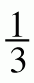x ≥ 2 c. 9(x − 2) > 18 d. 5 −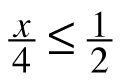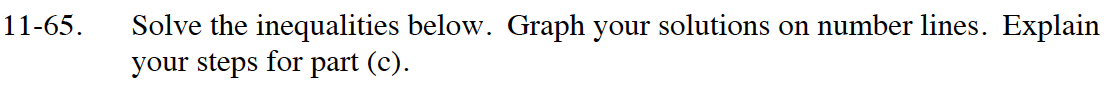Look at problem 11-21 in Lesson 11.1.2, which is similar to this problem.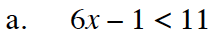Solve as an equation to find the boundary point.
Then check points in each region to determine which region is the solution.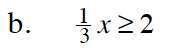Multiply by 3 to cancel out the fraction.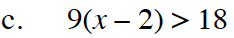Distribute and then solve.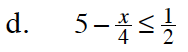Multiply by a common factor to cancel out the fractions.